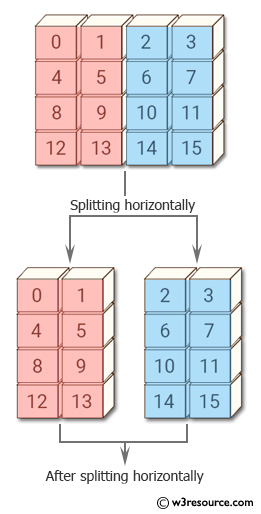﻿ NumPy: Split of an array of shape 4x4 it into two arrays along the second axis - w3resource# NumPy: Split of an array of shape 4x4 it into two arrays along the second axis

## NumPy: Array Object Exercise-62 with Solution

Write a NumPy program to split of an array of shape 4x4 it into two arrays along the second axis.
Sample array :
[[ 0 1 2 3]
[ 4 5 6 7]
[ 8 9 10 11]
[12 13 14 15]]

Pictorial Presentation:Sample Solution:

Python Code:

``````import numpy as np
x = np.arange(16).reshape((4, 4))
print("Original array:",x)
print("After splitting horizontally:")
print(np.hsplit(x, [2, 6]))
```
```

Sample Output:

```Original array: [[ 0  1  2  3]
[ 4  5  6  7]
[ 8  9 10 11]
[12 13 14 15]]
After splitting horizontally:
[array([[ 0,  1],
[ 4,  5],
[ 8,  9],
[12, 13]]), array([[ 2,  3],
[ 6,  7],
[10, 11],
[14, 15]]), array([], shape=(4, 0), dtype=int64)]
```

Python Code Editor:

Have another way to solve this solution? Contribute your code (and comments) through Disqus.

What is the difficulty level of this exercise?

Test your Python skills with w3resource's quiz

﻿

## Python: Tips of the Day

Set comprehension:

```>>> m = {x ** 2 for x in range(5)}
>>> m
{0, 1, 4, 9, 16}
```